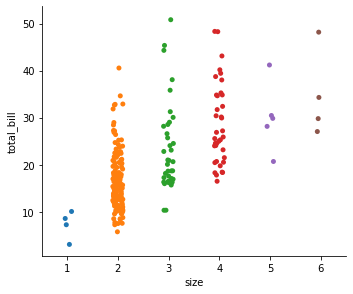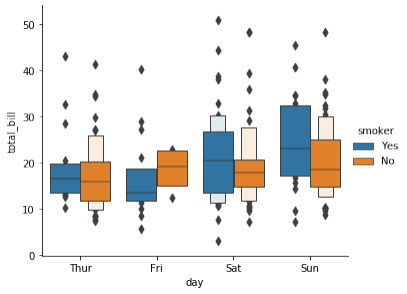Data Science using Python Tutorials

# Visualizing categorical data using Seaborn

In the relational plot tutorial, we saw how to use different visual representations to show the relationship between multiple variables in a  dataset. In the examples, we focused on cases where the main relationship was between two numerical variables. If one of the main variables is categorical ( divided into discrete groups ), it may be helpful to use a more specialized approach to visualization.

In seaborn, there are several different ways to visualize a relationship involving categorical data. Similar to the relationship between relplot() and either scatterplot() or lineplot(), there are two ways to make these plots. There are several axes-level functions for plotting categorical data in different ways and a figure-level interface, catplot(), that gives unified higher-level access to them.

It’s helpful to think of the different categorical plot kinds as belonging to three different families, which we’ll discuss in detail below.

Categorical scatterplots:

• stripplot() (with kind=”strip”; the default)

• swarmplot() (with kind=“swarm”)

Categorical distribution plots:

• boxplot() (with kind=“box”)

• violinplot() (with kind=“violin”)

• boxenplot() (with kind=“boxen”)

Categorical estimate plots:

• pointplot() (with kind=“point”)

• barplot() (with kind=“bar”)

• countplot() (with kind=”count”)

These families represent the data using different levels of granularity.  When deciding which to use, you’ll have to think about the question  that you want to answer.

The unified API makes it easy to switch between different kinds and see your data from several perspectives. In this tutorial, we’ll mostly focus on the figure-level interface, catplot(). Remember that this function is a higher-level interface each of the functions above, so we’ll reference them when we show each kind of plot, keeping the more verbose kind-specific API documentation at hand. We will use the tips data set.

```import seaborn as sns
import matplotlib.pyplot as plt  tips = sns.load_dataset(“tips”) ```

total_bill tip sex smoker day time size 0 16.99 1.01 Female No Sun Dinner 2 1 10.34 1.66 Male No Sun Dinner 3 2 21.01 3.50 Male No Sun Dinner 3 3 23.68 3.31 Male No Sun Dinner 2 4 24.59 3.61 Female No Sun Dinner 4

Categorical scatterplots

The default representation of the data in catplot() uses a scatterplot.  There are two different categorical scatter plots in seaborn. They take different approaches to resolving the main challenge in representing categorical data with a scatter plot, which is that all of the points belonging to one category would fall on the same position along with the axis corresponding to the categorical variable.  The approach used by strip plot(), which is the default kind in catplot() is to adjust the positions of points on the categorical axis with a small amount of random jitter.

`sns.catplot(x="day", y="total_bill", data=tips)`

The jitter parameter controls the magnitude of jitter or disables it  altogether.

`sns.catplot(x="day", y="total_bill", data=tips,  jitter=False)`

The second approach adjusts the points along the categorical axis using an algorithm that prevents them from overlapping. It can give a better representation of the distribution of observations, although it only works well for relatively small datasets. This kind of plot is sometimes called a  bees-warm and is drawn in seaborn by swarmplot(), which is activated by setting kind= “swarm” in catplot().

`sns.catplot(x="day", y="total_bill", kind="swarm",  data=tips)`

Similar to the relational plots, it’s possible to add another dimension to a categorical plot by using a hue semantic. The categorical plots do not currently support size or style semantics.

Each different categorical plotting function handles the hue semantic differently. For the scatter plots, it is only necessary to change the color of the points.

`sns.catplot(x="day", y="total_bill", hue=“sex",  kind="swarm", data=tips)`

Unlike with numerical data, it is not always obvious how to order the levels of the categorical variable along its axis. In general, the seaborn categorical plotting functions try to infer the order of categories from the data. If your data have a panda’s Categorical data using Seaborn type, then the default order of the categories can be set there. If the variable passed to the categorical axis looks numerical, the levels will be sorted. But the data are still treated as categorical and drawn at ordinal positions on the categorical axes even when numbers are used to label them.

`sns.catplot(x="size", y="total_bill", data=tips)`

The other option for choosing a default ordering is to take the levels of  the category as they appear in the dataset. The ordering can also be  controlled on a plot-specific basis using the order parameter. This can  be important when drawing multiple categorical plots in the same  figure, which we’ll see more of below.

`sns.catplot(x="smoker", y="tip", order=["No", “Yes”],  data=tips)  `

## Distributions of observations within categories

As the size of the dataset grows, categorical scatter plots become  limited in the information they can provide about the distribution of  values within each category. When this happens, there are several  approaches for summarizing the distributional information in ways that  facilitate easy comparisons across the category levels.

Boxplots

The first is the familiar boxplot(). This kind of plot shows the three  quartile values of the distribution along with extreme values. The  whiskers extend to points that lie within 1.5 IQRs of the lower and  upper quartile, and then observations that fall outside this range are  displayed independently. This means that each value in the boxplot  corresponds to an actual observation in the data.

`sns.catplot(x="day", y="total_bill", kind=“box",  data=tips) `

When adding a hue semantic, the box for each level of the semantic  variable is moved along the categorical axis so they don’t overlap.

`sns.catplot(x="day", y="total_bill", hue=“smoker",  kind="box", data=tips)`

This behavior is called “dodging” and is turned on by default because it  is assumed that the semantic variable is nested within the main  categorical variable.

A related function, boxenplot(), draws a plot that is similar to a box  plot but optimized for showing more information about the shape of the  distribution. It is best suited for larger datasets.

`sns.catplot(x="day", y="total_bill", kind=“boxen",  data=tips,hue=‘smoker') `Violinplots

A violin plot plays a similar role as a box and whisker plot. It shows the  distribution of quantitative data across several levels of one or more  categorical data using seaborn variables such that those distributions can be compared.  Unlike a box plot, in which all of the plot components correspond to  actual datapoints, the violin plot features a kernel density estimation of  the underlying distribution.

This can be an effective and attractive way to show multiple  distributions of data at once, but keep in mind that the estimation  procedure is influenced by the sample size, and violins for relatively  small samples might look misleadingly smooth.

`sns.catplot(x="day", y="total_bill", kind=“violin",  data=tips) `

This approach uses the kernel density estimate to provide a richer  description of the distribution of values. Additionally, the quartile and  whisker values from the boxplot are shown inside the violin.

We can also possible to “split” the violins when the hue parameter has  only two levels, which can allow for a more efficient use of space

`sns.catplot(x="day", y="total_bill", kind="violin",  data=tips,hue='smoker') `

It can also be useful to combine swarmplot() or striplot() with a  box plot or violin plot to show each observation along with a summary of  the distribution

`g = sns.catplot(x="day", y=“total_bill",  kind="violin", inner=None, data=tips)  `
`sns.swarmplot(x="day", y="total_bill", color=“k",  size=3, data=tips, ax=g.ax) `

In the next article we will learn how to plot statistical estimation within  categories along with joint and pair plots